# force

forceClass XChapter 1 – ForcePhysics

______________________________________________________________________________

EXERCISE- 1 (A)

Question 1:

What are contact forces? Give two Examples.

Solution 1:

The forces which act on bodies when they are in physical contact, are called contact forces. For e.g. frictional force and force exerted on two bodies during collision.

Question 2:

What are non – contact forces? Give two example.

Solution 2:

The forces experienced by bodies even without being physically touched, are called the non- contact forces. For e.g. Gravitational force and Electrostatic force.

Question 3:

Classify the following amongst contact and non-contact forces.

(a)Frictional force

(b)normal reaction force,

(c)force of tension in a string

(d)gravitation force

(e)electrostatic force

(f)magnetic force

Solution 3:

Contact force: (a) frictional force (b) normal reaction force (c) force of tension in a string

Non-contact force: (d) gravitational force (e) electric force (f) magnetic force

Question 4:

Give one example in each case where:

(a)the force is of contact and

(b)Force is at a distance.

Solution 4:

(a)Force exerted on two bodies during collision.

(b)Magnetic force between magnetic poles.

bpscccrackecr.com bpscccrackecr.com/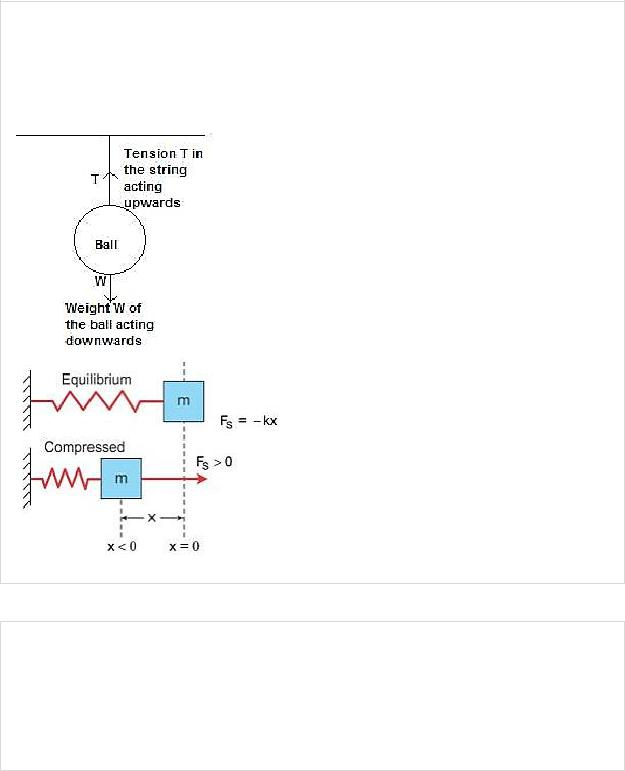Class XChapter 1 – ForcePhysics

______________________________________________________________________________

Question 5:

(a)A ball is hanging by a thread from the ceiling of the roof. Draw a neat labelled diagram showing the forces acting on the ball and the string.

(b)A spring is compressed against a rigid wall. Draw a neat and labelled diagram showing the forces acting on the spring.

Solution 5:

Question 6:

State one factor on which the magnitude of a non – contact force depends. How does it depend on the factor stated by you?

Solution 6:

The magnitude of a non-contact force depends on distance of separation of two bodies. Magnitude of force decreases as the distance increases.

bpscccrackecr.com bpscccrackecr.com/Class XChapter 1 – ForcePhysics

______________________________________________________________________________

Question 7:

The separation between two masses is reduced to half. How is the magnitude of gravitational force between them affected?

Solution 7:

The magnitude of gravitational force between two masses will become four times as gravitational force varies inversely as the square of distance of separation.

Question 8:

Define the term ‘force’?

Solution 8:

A Force is that physical cause which changes(or tends or change) either the size or shape or the state of rest or motion of the body.

Question 9:

State the effects of a force applied on (i) a non- rigid, and (ii) a rigid body. How does the effect of the force differ in the two cases?

Solution 9:

A force when applied on a non-rigid body changes the inter-spacing between its constituent particles and therefore causes a change in its dimensions and can also produce motion in it.

On the other hand, a force when applied on a rigid body, does not change the inter-spacing between its constituent particles and therefore it does not change the dimensions of the body but causes motion in it.

Question 10:

Give one example in each of the following cases:

(a)A force stops a moving body

(b)A force moves a stationary body

(c)A force changes the size of a body

(d)A force changes the shape of a body

Solution 10:

(a)A fielder on the ground stops a moving ball by applying a force with his hands.

(b)The pull exerted by horse makes a cart moves.

(c)In a cycle pump, when the piston is lowered, the air is compressed to occupy a less volume.

(d)On pressing a piece of rubber, its shape changes.

bpscccrackecr.com bpscccrackecr.com/∆𝑡 ∆𝑡

Class XChapter 1 – ForcePhysics

_____________________________________________________________________________

Question 11:

State Newton’s first law of motion. Why is it called the law of inertia?

Solution 11:

Newton’s first law of motion: A body continues to be in its state of rest or of uniform motion in a straight line unless an external force is applied on it. It is called the law of inertia because it tells that every material body has a property by virtue of which it resists the change in its state of rest or in its state of motion. This property is called inertia.

Question 12:

Define the term linear momentum. State its S.I. unit.

Solution 12:

The product of mass and velocity of the body is called linear momentum. S.I. unit of linear momentum is kg m s-1.

Question 13:

(a)Write an expression for the change in momentum of a body of mass m moving with velocity v if (i) v c and (ii) v c.

(b)State the condition when the change in the momentum of a body depends only on the change in its velocity.

Solution 13:

(i)When v <c,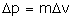.

(ii)When v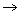c, the change in momentum is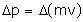.

If velocity v of the moving body is much smaller than the velocity of light c (v<c)

Question 14:

How is force related to the momentum of a body?

Solution 14:

The rate of change of momentum of a body is directly proportional to the force applied on it and this change in momentum takes place in the direction of the applied force, i.e.,

𝐹 ∝ ∆𝑝 ∆𝑃

Where p denotes momentum and ∆p is the change in momentum in time ∆t.

Question 15:

State Newton’s Second law of motion. Under what condition does it take the form F = ma?

Solution 15:

bpscccrackecr.com bpscccrackecr.com/Class XChapter 1 – ForcePhysics

______________________________________________________________________________

Newton’s second law of motion – The rate of change of momentum of a body is directly proportional to the force applied on it and this change in momentum takes place in the direction of the applied force, i.e.,

F∆𝑃∆𝑡

where p denotes momentum and ∆p is the change in momentum in time ∆t.

When mass m of the body is constant at velocity v (which is much smaller than the velocity of light c) then rate of change of momentum is:

F =∆𝑃∆𝑡 = m ∆𝑣∆𝑡 = ma

F= ma

Question 16:

Complete the following sentences:

(a)Mass X change in velocity = ….. x time interval.

(b)The mass of a body remains constant till the velocity of body is……

Solution 16:

(a)Mass X change in velocity = Force x time interval.

(b)The mass of a body remains constant till the velocity of body is Much less than the velocity of light.

Question 17:

Prove the force = mass x acceleration. State the condition when it holds.

Solution 17:

The rate of change of momentum = ∆𝑝 = ∆ (𝑚𝑣)

∆𝑡 ∆𝑡

(When vc or m is not constant). But if mass m is constant i.e., v<c

rate of change of momentum = ∆𝑝∆𝑡 = 𝑚 ∆𝑣∆𝑡

Here the quantity ∆𝑣∆𝑡= rate of change of velocity i.e., acceleration a.

Rate of change in momentum = ∆𝑝∆𝑡 = 𝑚 ∆𝑣∆𝑡 = 𝑚𝑎 Thus, by Newton’s second law of motion,

𝐹 ∝ 𝑚𝑎 or, F= k ma

Where k is a constant of proportionality which can be made equal to 1 by choosing the suitable unit for force.

Hence, F = ma when mass m of the body is constant at velocity v which is much smaller than the velocity of light.

bpscccrackecr.com bpscccrackecr.com/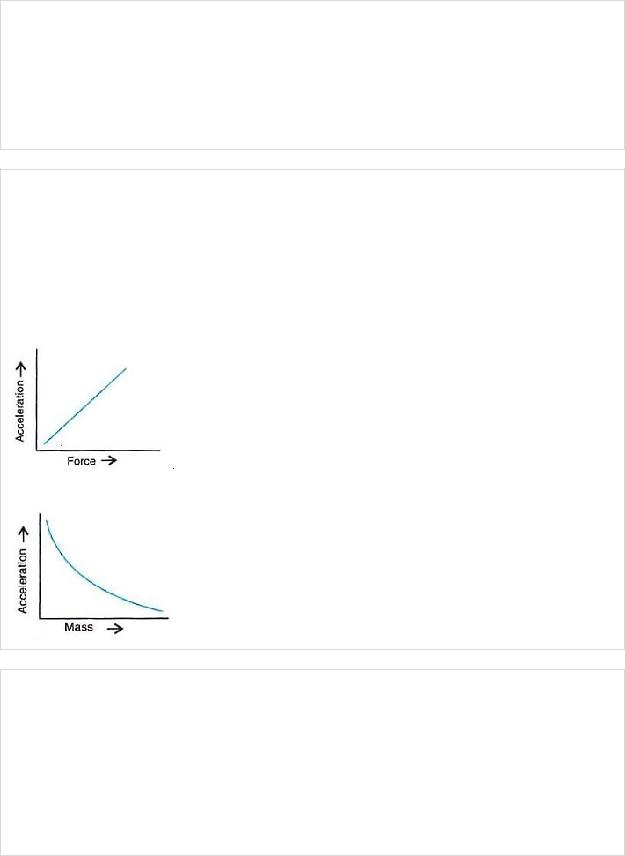Class XChapter 1 – ForcePhysics

______________________________________________________________________________

Question 18:

Name the S.I. unit of (a) momentum, (b) rate of change in momentum.

Solution 18:

S.I unit of

(a)momentum is kg m s-1

(b)rate of change in momentum is newton(N).

Question 19:

State the relationship between force, mass and acceleration. Draw graphs showing the relationship between:

(a)Acceleration and force for a constant mass.

(b)Acceleration and mass for a constant force.

Solution 19:

Force, F = mass (m) × Acceleration (a)

(b)

Question 20:

A rocket is moving at a constant speed in space by burning its fuel and ejecting out the burnt gases through a nozzle. Answer the following:

(a)Is there any change in the momentum of the rocket? If yes, what causes the change in momentum?

(b)Is there any force acting on the rocket? If yes, how much?

Solution 20:

(a)Yes, there is a change in momentum of the rocket. Decrease in mass of the rocket causes the change in momentum.

bpscccrackecr.com bpscccrackecr.com/Class XChapter 1 – ForcePhysics

______________________________________________________________________________

(b) Yes, there is force acting on the rocket which is equal to the rate of change in momentum.

Question 21:

State Newton’s third law of motion.

Solution 21:

Third law of motion states that –To every action, there is always an equal and opposite reaction.

Question 22:

Name and define the S.I. and C.G.S. unit of force. How are they related?

Solution 22:

S.I. unit of force is Newton (N).

One Newton is that force which when acting on a body of mass 1kg, produces an acceleration of 1 m s-2 in it.

C.G.S. unit of force is dyne.

One dyne is that force which when acting on a body of mass 1 gram, produces an acceleration of 1 cm s-2 in it.

1 Newton = 105 dyne

Question 23:

Define newton (the S.I. unit of force).

Solution 23:

S.I unit of force is newton (N).

One Newton is that force which when acting on a body of mass 1kg, produces an acceleration of 1 m s-2 in it.

Question 24:

Define 1 kgf how is it related to newton?

Solution 24:

One kilogram force is the force by which the Earth pulls a mass of 1 kilogram towards itself. 1 kgf = 9.8 N

Question 25:

Explain what is understood by the following statement: 1 kilogram force (kgf) = 9.8 newton’

bpscccrackecr.com bpscccrackecr.com/Class XChapter 1 – ForcePhysics

______________________________________________________________________________

Solution 25:

One kilogram force is the force by which the Earth pulls a mass of 1 kilogram towards itself. 1 kgf = 9.8 N

Question 26:

How can you feel a force of 1 N?

Solution 26:

It means that we have to exert a force of 9.8 N to hold a mass of 1 kg on our palm.

Question 27:

Complete the following:

(a)Force = mass x ……..

(b)1 N = ……… dyne

(c)1 N = ………… kgf (approx.)

(d)newton is the unit of ……….

Solution 27:

(a)Force = mass x Acceleration

(b)1 N = 105 dyne

(c)1 N = 0.1 kgf (approx.)

(d)Newton is the unit of Force.

MULTIPLE CHOICE TYPE:

Question 1:

1.which of the following is not the force at a distance:

(a) electrostatic force

(b) gravitational force

(c) frictional force

(d) magnetic force.

Solution 1:

Frictional force

Hint: It is the force which comes in play when two bodies come in contact.

bpscccrackecr.com bpscccrackecr.com/Class XChapter 1 – ForcePhysics

______________________________________________________________________________

Question 2:

Newton’s second law of motion applicable in all conditions is:

(a)F = ∆𝑝∆𝑡

(B)F = ma

(C)F = 𝑀 ∆𝑉∆𝑡

(d) all three

Solution 2:

F = ∆𝑝∆𝑡

NUMERICALS:

Question 1:

A body of mass 1 kg is thrown vertically up with an initial speed of 5 m s-1. What is the magnitude and direction of force due to gravity acting on the body when it is at its highest point? Take G = 9.8 n kg-1

Solution 1:

Mass of body= 1 kg Initial speed = 5 m/s

Acceleration due to gravity = 9.8 N/kg (vertically downwards) Force= mass x acceleration

=1 × 9.8 = 9.8N = 1 kgf

9.8 N or 1 kgf force acts vertically downwards.

Question 2:

A body of mass 1.5 kg is dropped from a height of 12m. What is the force acting on it during its fall? (g = 9.8 m s-2)

Solution 2:

Mass of body = 1.5 kg

Acceleration due to gravity = 9.8 ms-2

Force = mass (m) x acceleration due to gravity (g) = m g

F = 1.50 × 9.8 = 14.7 N

bpscccrackecr.com bpscccrackecr.com/Class XChapter 1 – ForcePhysics

______________________________________________________________________________

Question 3:

Two balls of masses in ratio 1:2 are dropped from the same height find:

(a)the ratio between their velocities when they strike the ground, and

(b)the ratio of the forces acting on them during motion.

Solution 3:

(a)Initial velocity, u = 0 m/s Final velocity = v

M1 : M2 =1 : 2

From equation: v2-u2 = 2gh So, v = √2𝑔ℎ

𝑣1 = √2𝑔ℎ = 1 = 1 ∶ 1 𝑣2 √2𝑔ℎ 1

(b) Force = mg

𝐹1 = 𝑚1𝑔 = 1 = 1: 2 𝐹2 𝑚2𝑔 2

Question 4:

A body X of mass 5 kg is moving with velocity 20 m s-1 while another body Y of mass 20 kg is moving with velocity 5 m S-1. Compare the momentum of the two bodies.

Solution 4:

Mass of body X = 5 kg

Velocity of body X = 20 m/s

Momentum of body X = mass x velocity

P1= 5 × 20 =100 kgm/s

Mass of body Y = 20kg

Velocity of body Y = 5m/s

Momentum of body Y = mass x velocity

P2 = 20 × 5 = 100 kgm/s

 𝑝1 = 𝑚1 𝑣1 = 5×20 = 100 = 1: 1 𝑝 𝑚 𝑣 20×5 100 2 2 2

Question 5:

Calculate the acceleration produced in a body of mass 50g when acted upon by a force of 20 N.

Solution 5:

Mass= 50g= 0.05kg

Force, F= 20N

Force = mass x acceleration

bpscccrackecr.com bpscccrackecr.com/Class XChapter 1 – ForcePhysics

______________________________________________________________________________

=ma

a = 𝑚𝐹 = 020.05 = 400𝑚𝑠−2

Question 6:

A car of mass 600 kg is moving with a speed of 10 ms-1 while a scooter of mass 80kg is moving with a speed of 50 m s-1 (a) Compare their momentum (b) which vehicle will require more force to stop it in the (i) same interval of time (ii) same distance.

Solution 6:

Mass of the car, M = 600 kg Mass of the scooter, m = 80 kg Velocity of car, v = 10 m/s Velocity of Scooter = 50 m/s

(a) we know that

Momentum = mass × Velocity

 𝑚𝑜𝑚𝑒𝑛𝑡𝑢𝑚 𝑜𝑓 𝑐𝑎𝑟 = 𝑀𝑉 = (600)(10) = 3 𝑚𝑜𝑚𝑒𝑛𝑡𝑢𝑚 𝑜𝑓 𝑠𝑐𝑜𝑜𝑡𝑒𝑟 𝑚𝑣 (80)(50) 2

Thus, the required ratio is 3:2

(b)(i) we know that force = 𝑐ℎ𝑎𝑛𝑔𝑒 𝑖𝑛 𝑚𝑜𝑚𝑒𝑛𝑡𝑢𝑚 𝑐ℎ𝑎𝑛𝑔𝑒 𝑖𝑛 𝑡𝑖𝑚𝑒

Considering the time interval to be the same,

Force a momentum

The momentum of car (600 × 10 = 6000 kgm/s)

Is more than the momentum of scooter (80 × 50 = 4000 kgm/s)

The car will require more force to stop

(ii) If we have to stop the vehicle at the same distance,

Then the vehicle travelling with greater velocity will require more force to stop it. The velocity of scooter (= 50 m/s) is greater than the velocity of the car (= 10m/s), The scooter shall require more force to stop at the same distance.

Question 7:

How much acceleration will be produced in a body of mass 10 kg acted upon by a force of 2 kgf? (g = 9.8 ms-2)

Solution 7:

Mass of body = 10 kg

Force = 2 kgf =2 × 9.8 =19.6 N Force = mass × acceleration

= ma

bpscccrackecr.com bpscccrackecr.com/Class XChapter 1 – ForcePhysics

______________________________________________________________________________

a = 𝑚𝐹 = 1910.6 = 1.96 ms-2

Question 8:

Two bodies have masses in the ratio 3:4, when a force is applied on the first body, it moves with an acceleration of 6 ms-2. How much acceleration will the same force produce in the other body?

Solution 8:

Force = mass × acceleration = ma

Force F1 = F2

𝑚1𝑎1 = 𝑚2𝑎2

𝑚1 = 𝑎2 𝑚2 𝑎1

Given, 𝑚1 = 3

𝑚2 4

𝑎1 = 6ms-2

𝒂𝟐= 𝒎𝟏𝒂𝟏= 𝟑×𝟔

𝒎𝟐 𝟒

= 4.5ms-2

Question 9:

A cricket ball of mass 100 g strikes the hand of a player with a velocity of 20 ms-1 and is brought to rest in 0.01 s. calculate :

(i)the force applied by the hand of the player,

(ii)the acceleration of the ball

Solution 9:

(i)Mass of cricket ball, m =100g= 0.1kg

∆v = (v – u) = ( 0 – 20) = − 20m/s Time ∆t = 0.01s

F×∆t = ∆p (change in momentum)

F = ∆𝑝∆𝑡 = 𝑚∆𝑣∆𝑡 = − 0.1×200.01 = −200𝑁

(ii)F = mass X acceleration F = m a

a = 𝑚𝐹 = −2000.1 = −2000𝑚𝑠−2

bpscccrackecr.com bpscccrackecr.com/Class XChapter 1 – ForcePhysics

______________________________________________________________________________

Question 10:

A lead bullet of mass 20g, travelling with a velocity of 350 ms-1, comes to rest after penetrating 40 cm in a still target. Find :

(i)the resistive force offered by the target and

(ii)the retardation caused by it.

Solution 10:

Mass of bullet=20g =0.020kg Initial velocity, u=350m/s Final velocity, v=0m/s Distance, s=40cm=0.4m From equation: v2-u2=2as 02-(350)2= 2a x 0.4

Acceleration a = 350 ×350 = −1.53 × 105 𝑚𝑠−2

2 ×0.4

(i) Resistive force, F = mass x acceleration F= 0.020 × 1.53 × 105

=3062.5N

(ii)Retardation = -acceleration = 1.53 × 105ms-2

Question 11:

A body of mass 50g is moving with a velocity of 10 ms-1. It is brought to rest by a resistive force of 10 N. find:

(i)the retardation and

(ii)the distance that the body will travel after the resistive force is applied.

Solution 11:

Mass of body = 50 g = 0.050 kg

Initial velocity, u = 10 m/s

Final velocity, v = 0 m/s

Resistive force, F = 10 N

(i)Force, F = ma 10N = 0.050 × a

Acceleration, a = 010.05 = 200ms-2

Retardation = − acceleration = 200 ms-2

(ii)From equation, v2 – u2 = 2as 02 – (10)2 = 2 × (-200)× s

−100 = − 400x s

s = 100400 = 0.25𝑚 = 25𝑐𝑚

bpscccrackecr.com bpscccrackecr.com/Class XChapter 1 – ForcePhysics

______________________________________________________________________________

Question 12:

A uniform car of mass 500g travels with a uniform velocity of 25 m s-1 for 5 s. The brakes are then applied and the car is uniformly retarded and comes to rest in further 10 s calculate:

(a)the retardation,

(b)the distance which the car travels after the brakes are applied,

(c)The force exerted by the brakes.

Solution 12:

Mass of toy car = 500 g = 0.5 kg Initial velocity, u = 25m/s Final velocity, v = 0m/s

From equation: v = u + at 0 = 25 + a × 10 Acceleration, a = − 2.5ms-2

(a)Retardation = − acceleration = 2.5ms-2

(b)From equation : v2-u2 = 2 as

02-(25)2 = 2 × (-2.5)× s

625 = −5s

S = 6255 = 125𝑚

(c)Force exerted by brake, F = ma

F = 0.5 × (− 2.5)

= −1.25 N

Resistive force exerted by brakes = 1.25 N

Question 13:

A truck of mass 5 × 103kg starting from rest travels a distance of 0.5 km in 10 s when a force is applied on it calculate:

(a)the acceleration acquired by the truck and

(b)the force applied

Solution 13:

Mass of truck = 5000 kg

Initial velocity, u = 0 m/s

Distance, s = 0.5 km = 500 m

Time, t =10 s

(a)From equation : S = ut + 12 𝑎𝑡2

500 = 0 × 10 + 12 𝑎 × (10)2 500 = 50a

a = 50050 = 10 𝑚𝑠−2

(b) Force = mass × acceleration

bpscccrackecr.com bpscccrackecr.com/Class XChapter 1 – ForcePhysics

______________________________________________________________________________

F = 5000 × 10 = 5 × 104 N

Question 14:

A force of 10 kgf is applied on a body of mass 100 g initially at rest for 0.1s calculate:

(a)the momentum acquired by the body,

(b)the distance travelled by the body in 0.1s. Take g = 10 N kg-1

Solution 14:

Mass of body = 100 g = 0.1 kg

Initial velocity, u=0m/s

Time , ∆t = 0.1s

(a)Force x change in time = change in momentum Force, F = 10 kgf = 10 x 10 = 100 N

(1 kgf =10 N)

F × ∆t = ∆p (change in momentum) 100 × 0.1 = ∆p

∆p = 10 KG m/s

(b)F= 100 N

Mass = 100g = 0.1kg F=ma

100 = 0.1 × a

Acceleration, a = 1000 ms-2 From equation:

S = ut + 12 𝑎𝑡2

S = 0 × 0.1 + 12 × 1000 × 0.1 × 0.1

S = 5m

EXERCISE – 1(B)

Question 1:

State the condition when a force produces

(a)translational motion,

(b)rotational motion, in a body

Solution 1:

(a)When the body is free to move it produces translational motion.

(b)When the body is pivoted at a point, it produces rotational motion.

Question 2:

Define moment of force and state its S.I. unit.

bpscccrackecr.com bpscccrackecr.com/Class XChapter 1 – ForcePhysics

______________________________________________________________________________

Solution 2:

The moment of force is equal to the product of the magnitude of the force and the perpendicular distance of the line of action of force from the axis of rotation.

S.I. unit of moment of force is Newton metre (Nm).

Question 3:

Is moment of force a scalar or a vector?

Solution 3:

Vector

Question 4:

State two factor on which moment of force about a point depends.

Solution 4:

Moment of force about a point depends on the following two factors: (a)The magnitude of the force applied and,

(b)The distance of line of action of the force from the axis of rotation.

Question 5:

When does a body rotate? State one way to change the direction of rotation of a body. Give a suitable example to explain your answer.

Solution 5:

When the body is pivoted at a point, the force applied on the body at a suitable point rotates the body about the axis passing through the pivoted point.

The direction of rotation can be changed by changing the point of application of force. The given figure shows the anticlockwise and clockwise moments produced in a disc pivoted at its centre by changing the point of application of force F from A to B.

Question 6:

Write the expression for calculating the moment of force about a given a axis.

Solution 6:

Moment of force about a given axis = Force x perpendicular distance of force from the axis of rotation.

bpscccrackecr.com bpscccrackecr.com/Class XChapter 1 – ForcePhysics

______________________________________________________________________________

Question 7:

State one way to reduce the moment of given force about a given axis of rotation.

Solution 7:

Moment of force depends on the distance of line of action of the force from the axis of rotation. Decreasing the perpendicular distance from the axis reduces the moment of a given force.

Question 8:

What do you understand by the clockwise and anticlockwise moment of force? When is it taken positive?

Solution 8:

If the turning effect on the body is anticlockwise, moment of force is called anticlockwise moment and it is taken as positive while if the turning effect on the body is clockwise, moment of force is called clockwise moment and is taken negative.

Question 9:

Why is it easier to open a door by applying the force at the free end of it?

Solution 9:

It is easier to open a door by applying the force at the free end of it because larger the perpendicular distance, less is the force needed to turn the body.

Question 10:

The stone of hand flour grinder is provided with a handle near its rim. Give a reason.

Solution 10:

The stone of hand flour grinder is provided with a handle near its rim so that it can be rotated easily about the iron pivot at its centre by a small force applied at the handle.

Question 11:

It is easier to turn the steering wheel of a large diameter then that of a small diameter. Give reason.

Solution 11:

It is easier to turn the steering wheel of a large diameter than that of a small diameter because less force is applied on steering of large diameter which is at a large distance from the centre of rim.

bpscccrackecr.com bpscccrackecr.com/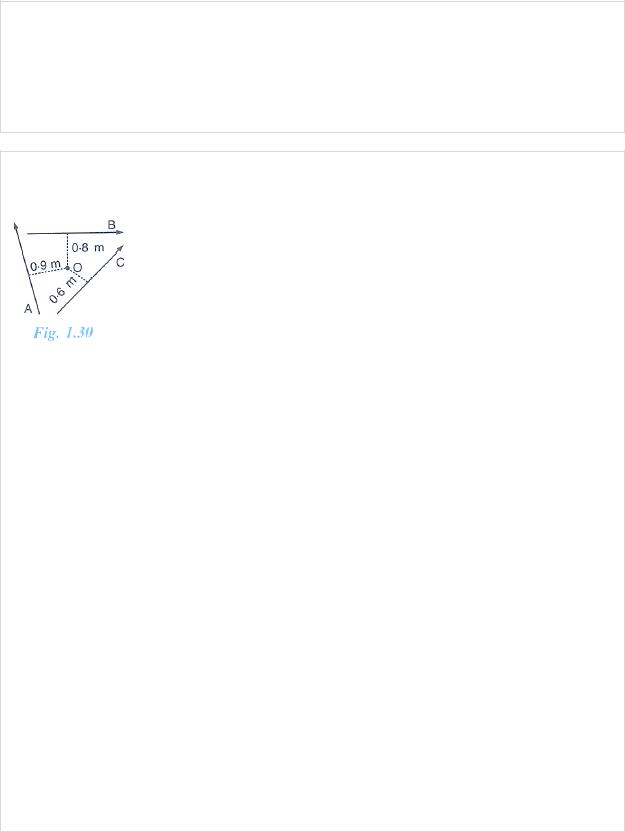Class XChapter 1 – ForcePhysics

______________________________________________________________________________

Question 12:

A spanner (or wrench) has a long handle. Why?

Solution 12:

A spanner (or wrench) has a long handle to produce larger turning moment so that nut can easily be turned with a less force.

Question 13:

A, B and C are the three forces each of magnitude 4 n acting in the plane of paper as shown in Fig. 1.30. The point O lies in the same plane.

(i)Which force has the least moment about O? Give a reason.

(ii)which force has the greatest moment about O? Give a reason.

(iii)Name the forces producing (a) Clockwise, (b) anticlockwise moments.

(iv)what is the resultant torque about the point O?

Solution 13:

(i) We know that,

Moment of force = Force × Perpendicular distance

Since the perpendicular distance of vector C is least from the point O, Vector C will have the least moment about O.

(ii) We know that,

Moment of force = Force × Perpendicular distance

Since the perpendicular distance of vector A is greatest from the point O, Vector A will have the greatest moment about O.

(iii)(a) Vector A and B will produce clockwise moments. Explanation: If the turning effect on the body is clockwise,

Moment if force is called clockwise moment and it is taken as negative.

(b)Vector C will produce anticlockwise moment. Explanation: if the turning effect on the body is anticlockwise,

Moment of force is called anticlockwise moment and it is taken as positive

(iv)Resultant torque about point O = sum of torques due to vectors A, B and C

=−(4 × 0.9) – (4 × 0.8) + (4×0.6) Nm

bpscccrackecr.com bpscccrackecr.com/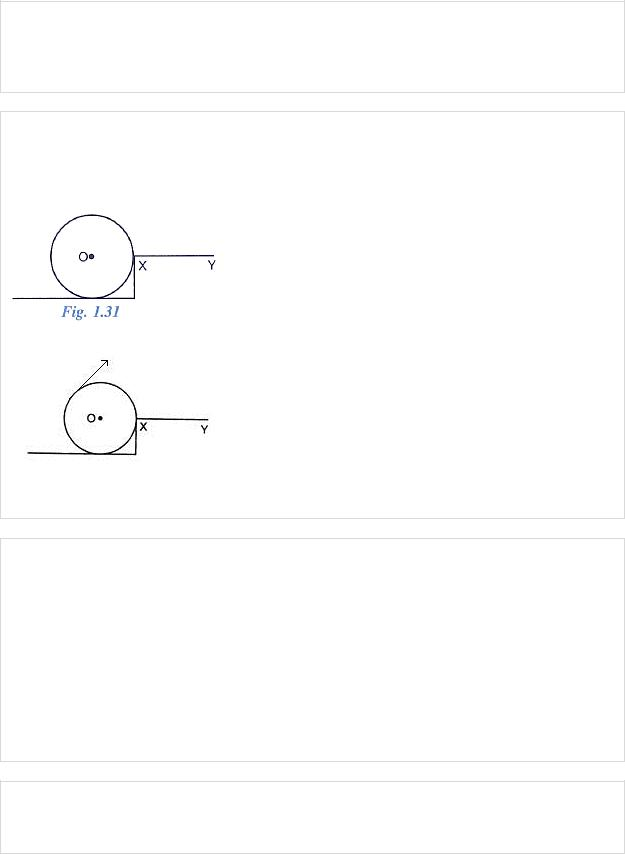Class XChapter 1 – ForcePhysics

______________________________________________________________________________

=− 3.6 – 3.2 + 2.4

=− 4.4 Nm

The negative sign suggests that the resultant torque is in the clockwise direction.

Question 14:

The adjacent diagram (Fig. 1.31) Shows a heavy roller, with its axle at O, which its axle at O, which is to be raised on a pavement XY by applying a minimum possible force. Show by an arrow on the diagram the point of application and the direction in which the force should be applied.

Solution 14:

Force F should be provided in the direction as shown in the diagram.

Question 15:

A body is acted upon by two forces each of magnitude F, but in opposite direction. State the effect of the forces if

(a)both forces act at the same point of the body.

(b)the two forces act at two different point of the body at a separation r.

Solution 15:

(a)Resultant force acting on the body = F − F = 0 moment of forces = 0 i.e., no motion of the body

(b)The forces tend to rotate the body about the mid-point between two forces, Moment of forces = Fr

Question 16:

Draw a neat labelled diagram to show the direction of two forces acting on a body to produce rotation in it. Also mark the point about which rotation takes place, by the letter O.

bpscccrackecr.com bpscccrackecr.com/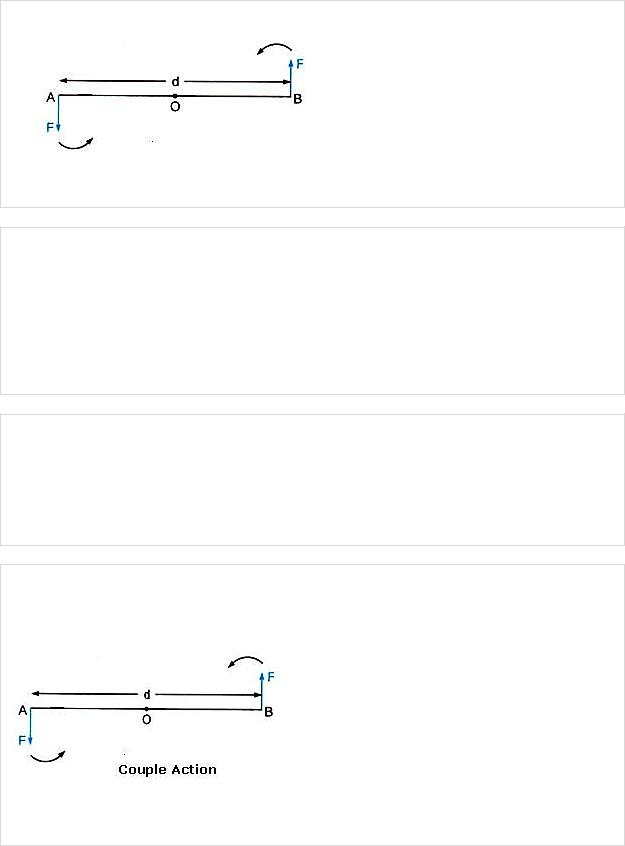Class XChapter 1 – ForcePhysics

______________________________________________________________________________

Solution 16:

At A and B, two equal and opposite forces each of magnitude F are applied. The two forces rotate the bar in anticlockwise direction.

Question 17:

What do you understand by the term couple? State its effect. Give two examples of couple action in our daily life.

Solution 17:

Two equal and opposite parallel forces not acting along the same line, form a couple. A couple is always needed to produce the rotation. For example, turning a key in a lock and turning a steering wheel.

Question 18:

Define moment of couple. Write its S.I. unit.

Solution 18:

The moment of a couple is equal to the product of the either force and the perpendicular distance between the line of action of both the forces. S.I unit of moment of couple is Nm.

Question 19:

Prove that

Moment of couple = Force × couple arm.

Solution 19:

At A and B, two equal and opposite forces each of magnitude F are applied. The two forces rotate the bar in anticlockwise direction. The perpendicular distance between two forces is AB

bpscccrackecr.com bpscccrackecr.com/Class XChapter 1 – ForcePhysics

______________________________________________________________________________

which is called the couple arm. Moment of force F at the end A

=F × OA (anticlockwise) Moment of force F at the end B

=F × OB (anticlockwise)

Total moment of couple =F × OA + F × OB

=F × (OA + OB) = F × AB

=F × d (anticlockwise)

=Either force x perpendicular distance between the two forces (or couple arm) Thus, Moment of couple = Force × Couple arm

Question 20:

What do you mean by equilibrium of a body?

Solution 20:

When a number of forces acting on a body produce no change in its state of rest or of motion, the body is said to be in equilibrium.

Question 21:

State the condition when a body is in (i) static (ii) dynamic, equilibrium. Give one example each of static and dynamic equilibrium.

Solution 21:

(i)When a body remains in the state of rest under the influence of the applied forces, the body is in static equilibrium. For example a book lying on a table is in static equilibrium.

(ii)When a body remains in the same state of motion (translational or rotational), under the influence of the applied forces, the body is said to be in dynamic equilibrium. For example, a rain drop reaches the earth with a constant velocity is in dynamic equilibrium.

Question 22:

State two condition for a body acted upon by several forces to be in equilibrium.

Solution 22:

For a body to be in equilibrium:

(i)The resultant of all the forces acting on the body should be equal to zero.

(ii)The resultant moment of all the forces acting on the body about the point of rotation should be zero.

Question 23:

State the principal of moments. Give one device as application of it.

bpscccrackecr.com bpscccrackecr.com/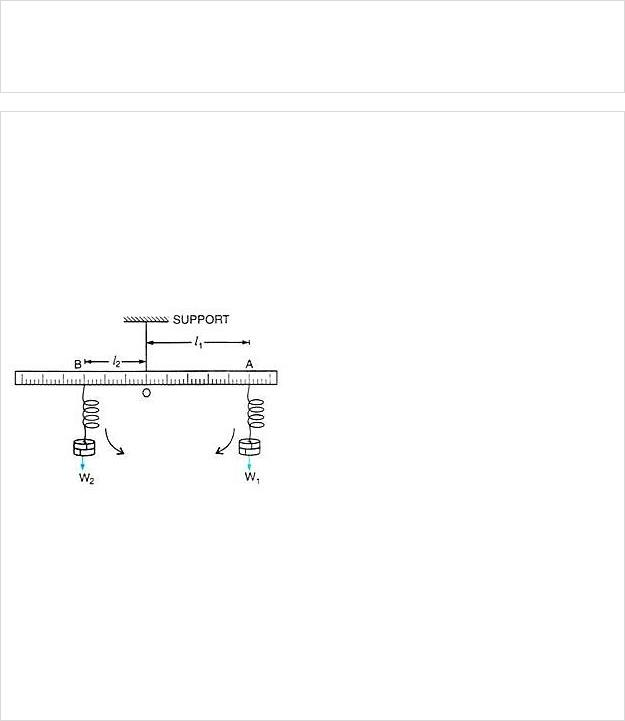Class XChapter 1 – ForcePhysics

______________________________________________________________________________

Solution 23:

According to the principle of moments, if the algebraic sum of moments of all the forces acting on the body about the axis of rotation is zero, the body is in equilibrium. A physical balance (or beam balance) works on the principle of moments.

Question 24:

Describe a simple experiment to verify the principle of moments, if you are supplied with a metre rule, a fulcrum and two springs with slotted weights.

Solution 24:

Suspend a metre rule horizontally from a fixed support by means of a strong thread at O as shown. Now suspend two spring balances with some slotted weights W1 and W2 on them on either side of the thread. The scale may tilt to one side. Now adjust the distances of two spring balances from the support by keeping one at A and the other at B in such a way that the scale again becomes horizontal.

Let the weight suspended on the right side of thread from the spring balance at A be W1 at distance OA = l1, while the weight suspended on the left side of thread from the spring balance at B be W2 at distance OB = l2.

The weight W1 tends to turn the scale clockwise, while the weight W2 tend to turn the scale anticlockwise.

Clockwise moment = W1 × l1 Anticlockwise moment = W2 × l2

In equilibrium, when the scale is horizontal, it is found that Clockwise moment = Anticlockwise moment

i.e., W1 × l1 = W2 × l2

This verifies the principle of moments.

bpscccrackecr.com bpscccrackecr.com/Class XChapter 1 – ForcePhysics

______________________________________________________________________________

MULTIPLE CHOICE TYPE:

Question 1:

The moment of a force about a given axis depends:

(a)only on the magnitude of force

(b)only on the perpendicular distance of force from the axis.

(c)neither on the force nor on the perpendicular distance of force from the axis

(d)both on the force and its perpendicular distance from the axis.

Solution 1:

The moment of a force about a given axis depends on both on the force and its perpendicular distance from the axis.

Hint: Moment of force = Force x Perpendicular distance

Question 2:

A body is acted upon by two unequal forces in opposite directions, but not in same line. The effect is that:

(a)the body will have only the rotational motion

(b)the body will have only the translational motion

(c)the body will have neither the rotational motion nor the translational motion.

(d)the body will have rotational as well as translational motion.

Solution 2:

(a) The body will have rotational as well as translational motion.

NUMERICALS

Question 1:

The moment of a force of 10 N about a fixed point O is 5 N m. Calculate the distance of the point O from the line of action of the force.

Solution 1:

Moment of force = force x perpendicular distance of force from point O Moment of force = F x r

5Nm = 10 × r

R = 5/10 = 0.5 m

Question 2:

A nut is opened by a wrench of length 10 cm. if the least force required is 5.0 N, find the moment of force needed to turn the nut.

Solution 2:

bpscccrackecr.com bpscccrackecr.com/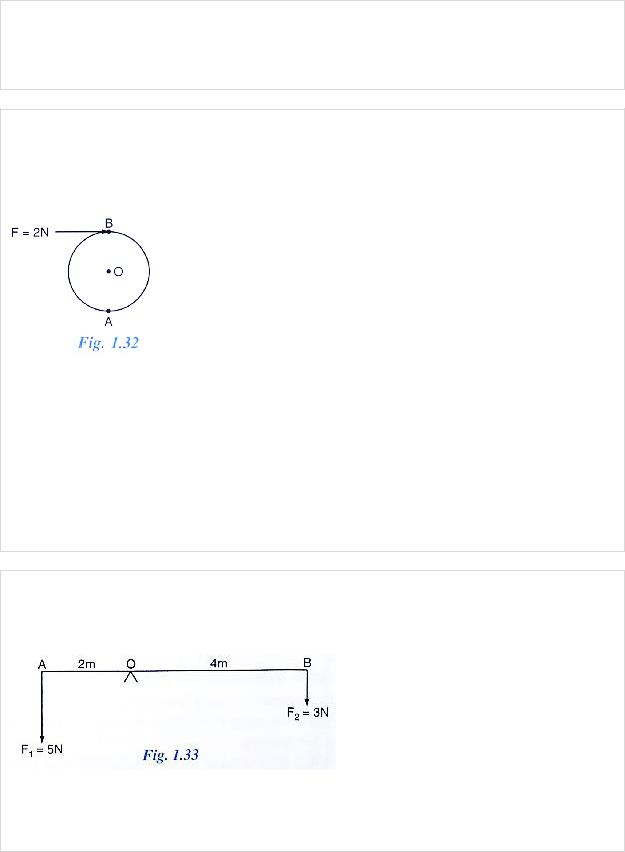Class XChapter 1 – ForcePhysics

______________________________________________________________________________

Length, r = 10 cm = 0.1 m

F= 5N

Moment of force = F × r = 5 × 0.1 = 0.5 Nm

Question 3:

A Wheel of diameter 2 m is shown in Fig. 1.32 with axle at O. A force F = 2 N is applies at B in the direction shown in figure. Calculate the moment of force about (i) Centre O, and

(ii) point A.

Solution 3:

Given , F = 2N

Diameter = 2m

Perpendicular distance between B and O = 1m

(i) Moment of force at point O = F x r

=2 x 1 = 2 Nm (clockwise)

(ii)Moment of force at point A = F × r =2 × 2 = 4 Nm (clockwise)

Question 4:

The diagram in fig.1.33 shows two forces F1 = 5 N and F2 = 3N acting at points A and B of a rod pivoted at a point O, such that OA = 2m and OB = 4m.

Calculate:

(i)Moment of force F1 about O.

(ii)Moment of force F2 about O.

(iii)Total moment of the two forces about O.

bpscccrackecr.com bpscccrackecr.com/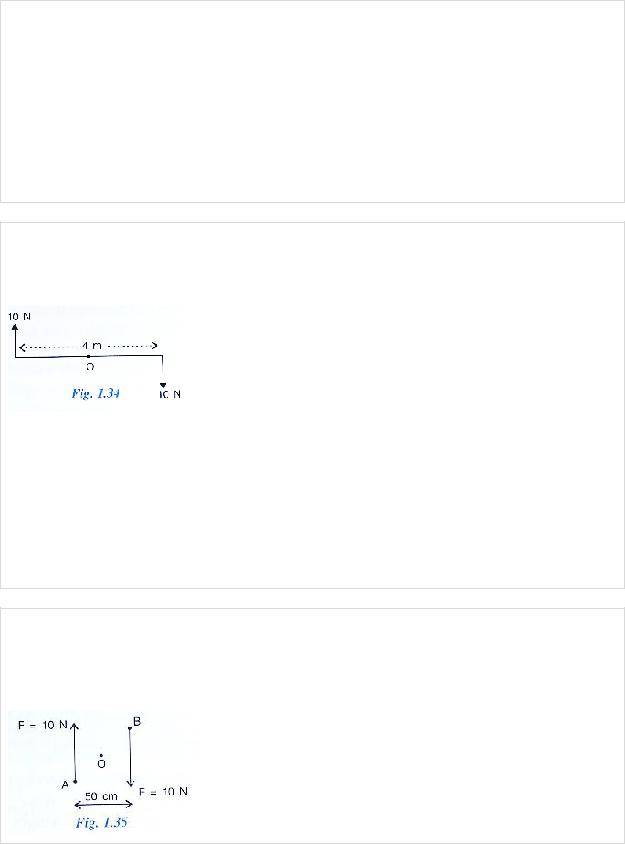Class XChapter 1 – ForcePhysics

______________________________________________________________________________

Solution 4:

Given AO = 2m and OB = 4m

(i)Moment of force F1(= 5N) at A about the point O

=F1 × OA

=5 × 2 = 10Nm (anticlockwise)

(ii)Moment of force F2 (= 3N) at B about the point O

=F2 × OB

=3 × 4 =12 Nm(clockwise)

(iii)Total moment of forces about the mid-point O =

=12 – 10 = 2 Nm (clockwise)

Question 5:

Two forces each of magnitude 10 N act vertically upwards and downwards respectively at the two ends of a uniform road of length 4 m which is pivoted at its mid point as shown in fig 1.34. Determine the magnitude of resultant moment of forces about the pivot O.

Solution 5:

Given, AB = 4 m hence, OA = 2m and OB = 2m Moment of force F (=10N) at A about the point O = F × OA = 10 × 2 = 20 Nm (clockwise)

Moment of force F (=10N) at point B about the point O = F × OB= 10 × 2 = 20 Nm (clockwise)

Total moment of forces about the mid-point O= = 20 + 20 = 40 Nm(clockwise)

Question 6:

Fig 1.35 shows two forces each of magnitude 10 N acting at the points A and B at a separation of 50 cm, in opposite directions. Calculate the resultant moment of the two forces about the point (i) A, (ii) B and (iii) O, situated exactly at the middle of the two forces.

bpscccrackecr.com bpscccrackecr.com/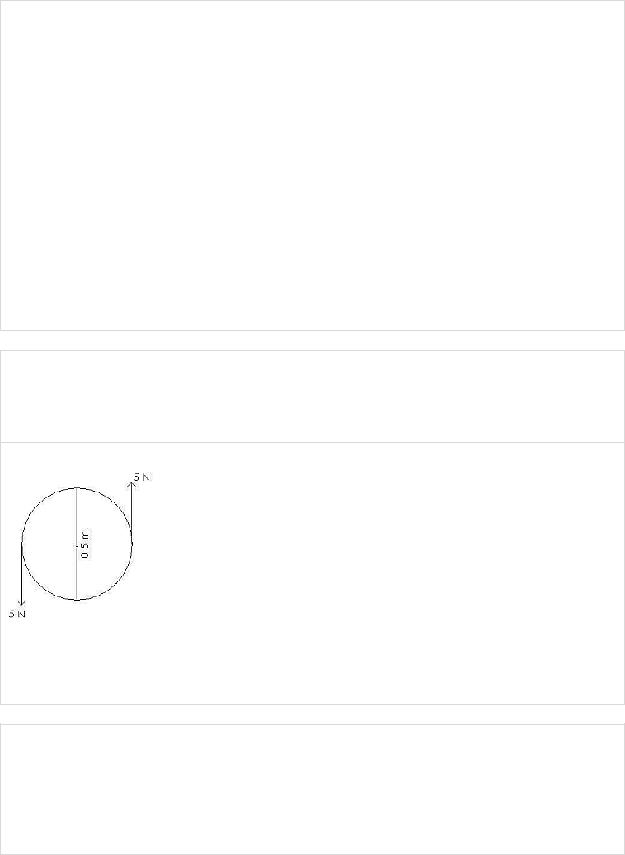Class XChapter 1 – ForcePhysics

______________________________________________________________________________

Solution 6:

(i)Perpendicular distance of point A from the force F = 10 N at B is 0.5m, while it is zero from the force F = 10 N at A

Hence, moment of force about A is

=10 N × 0.5m = 5 Nm(clockwise)

(ii)Perpendicular distance of point B from the force F = 10 N at A is 0.5m, while it is zero from the force F = 10 N at B

Hence, moment of force about B is

=10 N × 0.5m = 5Nm (clockwise)

(iii)Perpendicular distance of point O from either of the forces F = 10N is 0.25 m

Moment of force F (=10N) at A about O = 10N × 0.25m = 2.5 Nm(clockwise)

And moment of force F (=10N) at B about O = 10N × 0.25m=2.5 Nm(clockwise)

Hence, total moment of the two forces about O = 2.5 + 2.5 = 5 Nm (clockwise)

Question 7:

A steering wheel of diameter 0.5 m is rotated anticlockwise by applying two forces each of magnitude 5 N. Draw a diagram to show the application of forces and calculate the moment of couple applied.

Solution 7:

Moment of couple = either force × couple arm

=5 N × 0.5 m

=2.5 Nm

Question 8:

A uniform metre rule is pivoted at its mid-point. A weight of 50 gf is suspended at one end of it. Where should a weight of 100 gf be suspended to keep the rule horizontal?

Solution 8:

Let the 50 gf weight produce anticlockwise moment about the middle point of metre rule .i.e, at 50cm.

bpscccrackecr.com bpscccrackecr.com/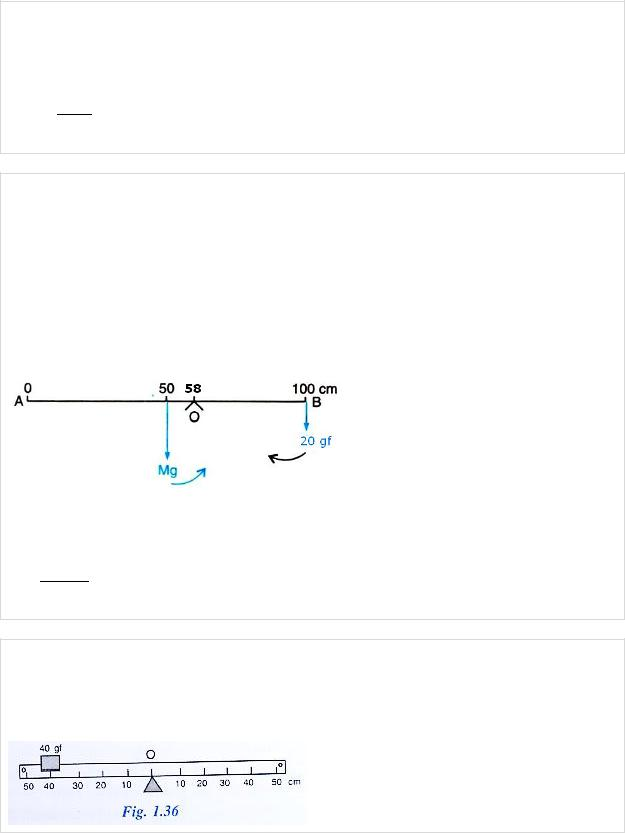Class XChapter 1 – ForcePhysics

______________________________________________________________________________

Let a weight of 100gf produce a clockwise moment about the middle point. Let its distance from the middle be d cm. Then,

according to principle of moments, Anticlockwise moment = Clockwise moment 50gf × 50 cm = 100gf × d

So, d = 50×50100 = 25cm from the other end

Question 9:

A uniform metre rule balance horizontally on a knife edge placed at the 58 cm mark when a weight of 20 gf is suspended from one end.

(i)Draw a diagram of the arrangement

(ii)What is the weight of the rule?

Solution 9:

(i)Weight mg (W) of rule produces an anti-clockwise moment about the knife edge O. In order to balance it, 20gf must be suspended at the end B to produce clockwise moment about the knife edge O.

(ii)From the principle of moments, Anticlockwise moment = Clockwise moment W × (58-50) = 20gf × (100 − 58)

W × 8 = 20gf × 42

W = 20gf × 42 = 105 gf

8

Question 10:

The diagram below (Fig 1.36) Shows a uniform bar supported at the middle point O. A weight of 40 gf is placed at a distance 40 cm to the left of the point O. How can you balance the bar with a weight of 80 gf?

bpscccrackecr.com bpscccrackecr.com/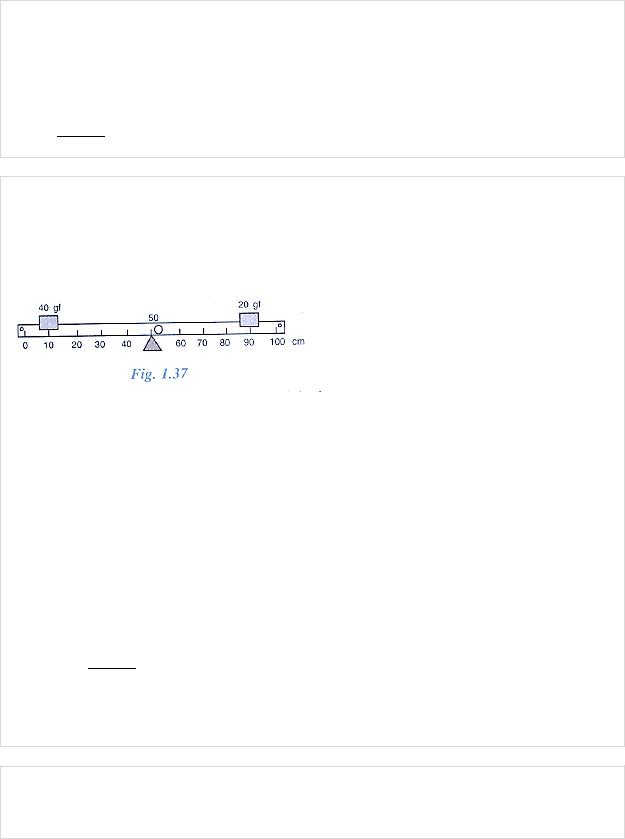Class XChapter 1 – ForcePhysics

______________________________________________________________________________

Solution 10:

Anticlockwise moment = 40gf × 40 cm Clockwise moment = 80 gf × d cm From the principle of moments, Anticlockwise moment = Clockwise moment 40 gf × 40 cm = 80 gf × d

So, d = 40gf ×40 = 20cm to the right of point O.

80

Question 11:

Fig 1.37 shows a uniform metre rule placed on a fulcrum at its mid-point O and having a weight 40 gf at the 10 cm mark and a weight of 20 gf at the 90 cm mark.

(i)Is the metre rule in equilibrium? If not, how will the rule turn?

(ii)How can the rule be brought in equilibrium by using an additional weight of 40 gf?

Solution 11:

(i)Anticlockwise moment = 40 gf × (50 − 10) cm =40gf × 40cm = 1600 gf × cm

Clockwise moment = 20gf × (90- 50) = 20 gf × 40cm =800 gf × cm

Anticlockwise moment is not equal to clockwise moment. Hence the metre rule is not in equilibrium and it will turn anticlockwise.

(ii)To balance it, 40gf weight should be kept on right hand side so as to produce a clockwise moment about the middle point. Let its distance from the middle be d cm. Then, clockwise moment = 20 gf × 40cm + 40gf × d cm

From the principle of moments, Anticlockwise moment= Clockwise moment 40 gf × 40 cm = 20gf × 40 + 40 × d cm 1600 – 800 = 40gf × dcm

So, d = 800gf cm = 20 cm (on the other side)

40gf

Hence, by placing the additional weight of 40 gf at the 70 cm mark the rule can be brought in equilibrium.

Question 12:

When a boy weighing 20 kgf sits at one end of a 4 m long see saw, it gets depressed at this end. How can it be brought to the horizontal position by a man weighing 40 kgf.

bpscccrackecr.com bpscccrackecr.com/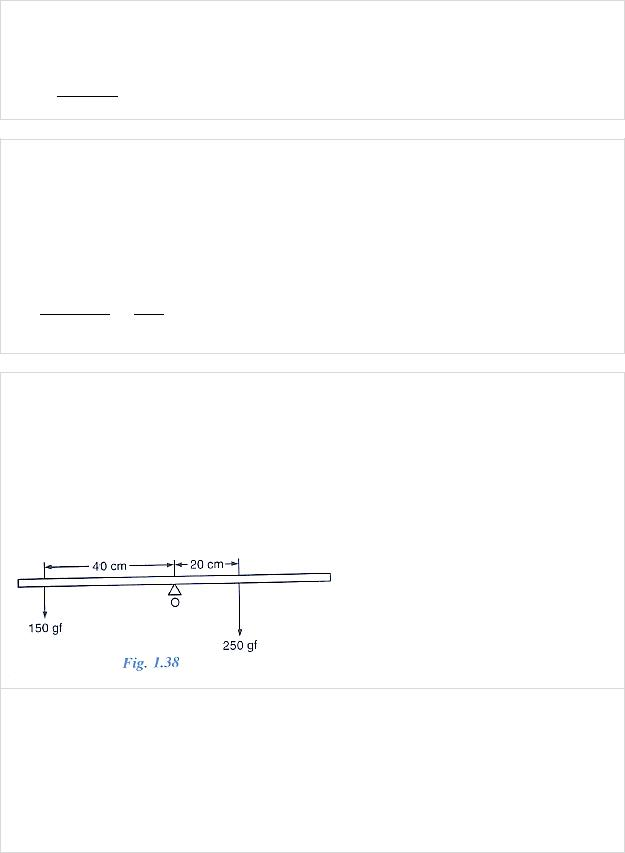Class XChapter 1 – ForcePhysics

______________________________________________________________________________

Solution 12:

From the principle of moments, Anticlockwise moment = Clockwise moment 20 kgf × 2m = 40kgf × d

So, d = 20 kgf ×2m = 1m from the centre on the side opposite to the boy.

40kgf

Question 13:

A physical balance has its arms of length 60 cm and 40 cm. what weight kept on pan of longer arm will balance an object of weight 100 gf kept on other pan?

Solution 13:

From the principle of moments, Anticlockwise moment = Clockwise moment 100 gf × 40 cm = W × 60 cm

So, weight on the longer pan ,

W = 100gf ×40cm = 200gf = 66.67 gf

60cm 3

Question 14:

The diagram in Fig 1.38 shows a uniform metre rule weighing 100gf, pivoted as its centre O. two weights 150 gf and 250 gf hang from the metre rule as shown.

Calculate:

(i)the total anticlockwise moment about o,

(ii)the total clockwise moment about O,

(iii)the difference of anticlockwise and clockwise moments, and

(iv)the distance from O where a 100 gf weight should be placed to balance the metre rule.

Solution 14:

=150 gf × 40 cm = 6000 gf cm

=250gf × 20 cm = 5000 gf cm

(iii) The difference of anticlockwise and clockwise moment = 6000 – 5000 = 1000gf cm

(iv)From the principle of moments, Anticlockwise moment = Clockwise moment

bpscccrackecr.com bpscccrackecr.com/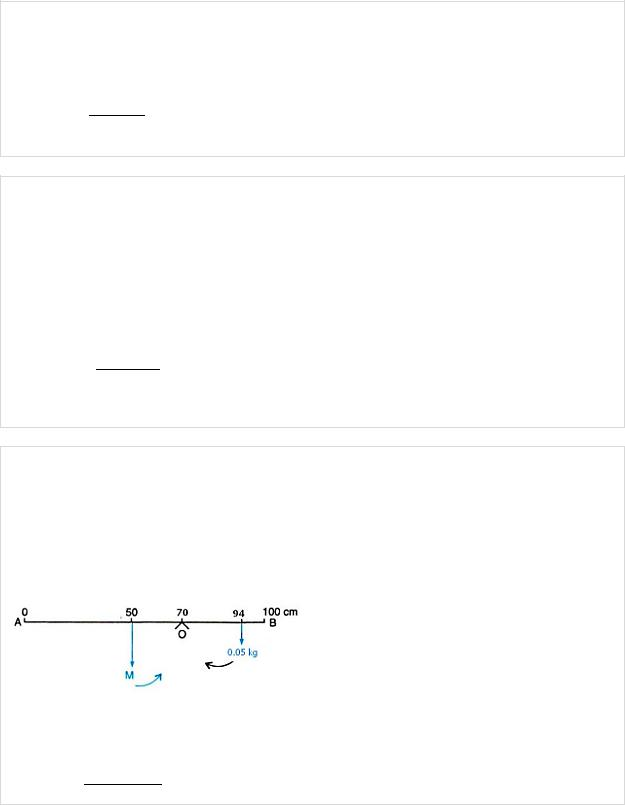Class XChapter 1 – ForcePhysics

______________________________________________________________________________

To balance it, 100gf weight should be kept on right hand side so as to produce a clockwise moment about the O. Let its distance from the point O be d cm. Then,

150gf × 40 cm = 250gf × 20 cm + 100gf × d 6000gf cm = 5000gf cm + 100gf × d 1000gf cm = 100 gf × d

So, d= 1000gf cm = 10 cm on the right side of O.

100gf

Question 15:

A uniform metre rule of weight 10gf is pivoted at its 0 mark.

(i)What moment of force depresses the rule?

(ii)How can it be made horizontal by applying a least force?

Solution 15:

(i)Anticlockwise moment= 10gf × 50 cm = 500gf cm

(ii)From the principle of moments,

Anticlockwise moment = Clockwise moment 10gf × 50 cm = W × 100cm

So, W = 10gf ×50cm = 5gf 100cm

By applying a force 5 gf upwards at the 100 cm mark, rule can be made horizontal

Question 16:

A uniform metre scale can be balanced at the 70.0 cm mark when a mass 0.05 kg is hung from the 94.0 cm mark.

(a)draw a diagram of the arrangement.

(b)Find the mass of the metre scale

Solution 16:

(b)From the principle of moments, Anticlockwise moment = Clockwise moment M × 20cm = 0.05kg × 24 cm

So, M = 0.05Kg ×24 cm = 0.06 kg

20 cm

bpscccrackecr.com bpscccrackecr.com/Class XChapter 1 – ForcePhysics

______________________________________________________________________________

Question 17:

A uniform metre rule of mass 100 g is balanced on a fulcrum at mark 10 cm by suspending an unknown mass M at the mark 20 cm.

(i)Find the value of M

(ii)To which side the rule will tilt if the mass m is moved to the mark 10cm?

(iii)what is the resultant moment now?

(iv)How can it be balanced by another mass of 50g?

Solution 17:

(i)From the principle of moments,

Clockwise moment = Anticlockwise moment 100g × (50 − 40) cm = m × (40 − 20) cm 100g × 10 cm = m × 20 cm = m = 50 g

(ii)The rule will tilt on the side of mass m (anticlockwise), if the mass m is moved to the mark 10cm.

(iii)Anticlockwise moment if mass m is moved to the mark 10 cm = 50g × (40−10)cm = 50 × 30 = 1500 g cm

Clockwise moment = 100g × (50 − 40) cm = 1000g cm

Resultant moment = 1500g cm − 1000g cm = 500g cm (anticlockwise)

(iv)From the principle of moments,

Clockwise moment = Anticlockwise moment

To balance it, 50g weight should be kept on right hand side so as to produce a clockwise moment. Let its distance from fulcrum be d cm. Then,

100g × (50 − 40) cm + 50g × d = 50g × (40 − 10)cm 1000g cm + 50g × d = 1500 g cm

50 g × d = 500g cm

So, d =10 cm

By suspending the mass 50g at the mark 50 cm, it can be balanced.

EXERCISE – 1 (C)

Question 1:

Define the term ‘centre of gravity of a body’.

Solution 1:

Centre of gravity is the point about which the algebraic sum of moments of weights of particles constituting the body is zero and the entire weight of the body is considered to act at this point.

Question 2:

Can the centre of gravity be situated outside the material of the body? Give an example.

Solution 2:

Yes, the centre of gravity can be situated outside the material of the body. For example, centre of gravity of ring.

bpscccrackecr.com bpscccrackecr.com/Class XChapter 1 – ForcePhysics

______________________________________________________________________________

Question 3:

On what factor does the position of centre of gravity of a body depend? Explain your answer with an example.

Solution 3:

The position of centre of gravity of a body of given mass depends on its shape i.e., on the distribution of mass in it. For example: the centre of gravity of a uniform wire is at its mid- point. But if this wire is bent into the form of a circle, its centre of gravity will then be at the centre of circle.

Question 4:

What is the position of centre of gravity of a:

(a)rectangular lamina

(b)cylinder?

Solution 4:

The position of centre of gravity of a

(a)rectangular lamina is at the point of intersection of its diagonals.

(b)cylinder is at the mid point on the axis of cylinder.

Question 5:

At which point is the centre of gravity situated in:

(a)a triangular lamina

(b)a circular lamina?

Solution 5:

(a)Centre of gravity of a triangular lamina is situated at the point of intersection of its medians.

(b)Centre of gravity of a circular lamina is situated at the centre of circular lamina.

Question 6:

Where is the centre of gravity of a uniform ring situated?

Solution 6:

Centre of gravity of a uniform ring is situated at the centre of ring.

bpscccrackecr.com bpscccrackecr.com/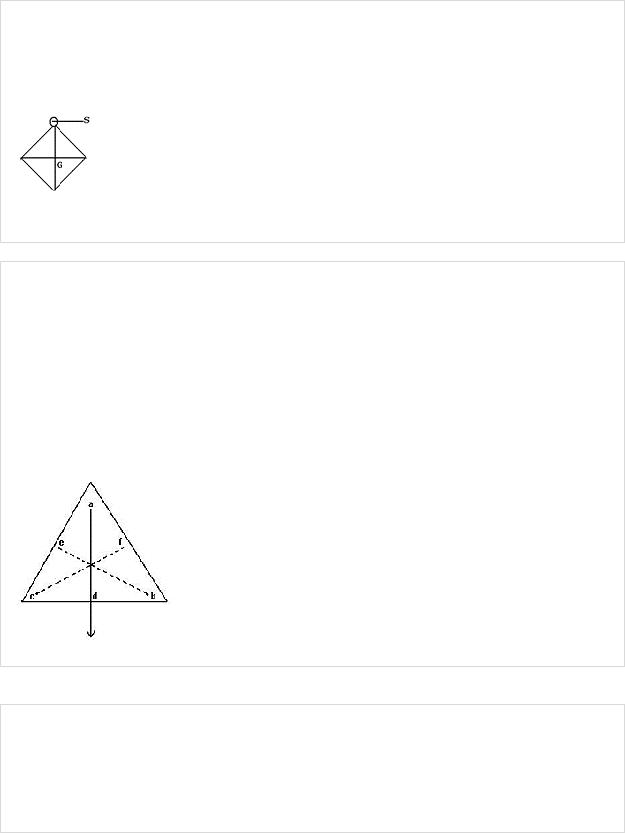Class XChapter 1 – ForcePhysics

______________________________________________________________________________

Question 7:

A square card board is suspended by passing a pin through a narrow hole at its one corner. Draw a diagram to show its rest position. In the diagram, mark the point of suspension by the letter S and centre of gravity by the letter G.

Solution 7:

A square card board in rest position with G as centre of gravity and S as point of suspension.

Question 8:

Explain how you will determine the position of centre of gravity experimentally for a triangular lamina (or a triangular piece of card board)

Solution 8:

Take a triangular lamina. Make three fine holes at a, b, c near the edge of triangular lamina. Now suspend the given lamina along with a plumb line from hole ‘a’. Check that the lamina is free to oscillate about the point of suspension. When lamina has come to rest, draw straight line ad along the plumb line. Repeat the experiment by suspending the lamina through hole ‘b’ and then through hole ‘c’ for which we get straight lines be and cf respectively. It is noticed that the lines ad, be and cf intersect each other at a common point G which is the position of centre of gravity of triangular lamina i.e. the point of intersection of medians.

Question 9:

State whether the following statements are true or false.

(i)‘The position of centre of gravity of a body remains unchanged even when the body is deformed’

(ii)‘Centre of gravity of a freely suspended body always lies vertically below the point of suspension’

bpscccrackecr.com bpscccrackecr.com/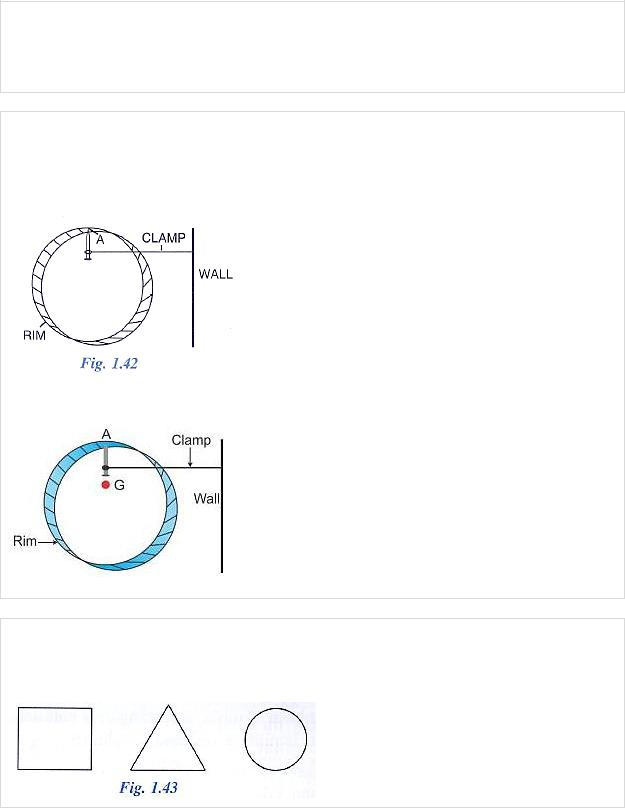Class XChapter 1 – ForcePhysics

______________________________________________________________________________

Solution 9:

(i)False. The position of centre of gravity of a body of given mass depends on its shape i.e., on the distribution of mass in it.

(ii)True.

Question 10:

A uniform flat circular rim is balanced on a sharp vertical nai by supporting it at point A, as shown in fig 1.42. Mark the position of centre of gravity of the rim in the diagram by the letter G.

Solution 10:

Question 11:

Fig. 1.43 shows three pieces of card board of uniform thickness cut into three different shapes. On each diagram draw two lines to indicate the position of centre of gravity G.

bpscccrackecr.com bpscccrackecr.com/